The SSM Procedure

Filtering, Smoothing, Likelihood, and Structural Break Detection

Subsections:

The Kalman filter and smoother (KFS) algorithm is the main computational tool for using SSM for data analysis. This subsection briefly describes the basic quantities generated by this algorithm and their relationship to the output generated by the SSM procedure. For proper treatment of SSMs with a diffuse initial condition or when regression variables are present, a modified version of the traditional KFS, called diffuse Kalman filter and smoother (DKFS), is needed. A good discussion of the different variants of the traditional and diffuse KFS can be found in Durbin and Koopman (2001). The DKFS implemented in the SSM procedure closely follows the treatment in De Jong and Chu-Chun-Lin (2003). Additional details can be found in these references.

The state space model equations (see the section State Space Model and Notation) imply that the combined response data vector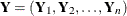has a Gaussian probability distribution. This probability distribution is proper if d, the dimension of the diffuse vector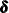in the initial condition, is 0 and if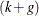, the total number of regression variables in the observation and state equations, is also 0 (the regression vectors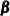and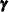are also treated as a diffuse vectors). Otherwise, this probability distribution is improper. The KFS algorithm is a combination of two iterative phases: a forward pass through the data, called filtering, and a backward pass through the data, called smoothing, that uses the quantities generated during filtering. One of the advantages of using the SSM formulation to analyze the time series data is its ability to handle the missing values in the response variables. The KFS algorithm appropriately handles the missing values in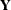. For additional information about how PROC SSM handles missing values, see the section Missing Values.